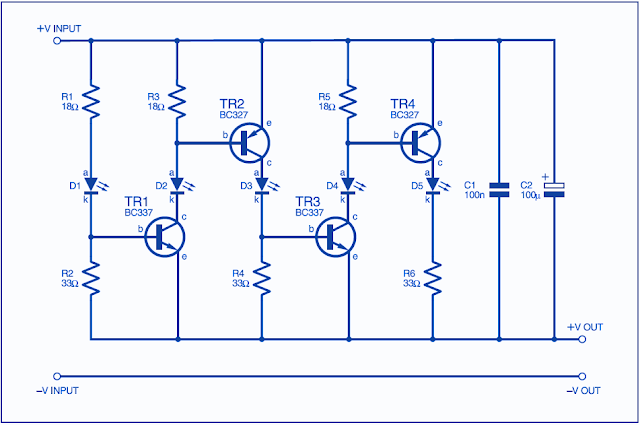## Feb 28, 2013

### BARGRAPH USING LED

The circuit shown in Fig was devised as a cheap alternative to a moving coil meter when I wanted to monitor the output current of a power supply. Its operation is as follows: As the output current increases from zero, it flows through l.e.d. D1 and resistors R1 and R2 until the voltage across R2 is about 0·6V. At this point transistor TR1 starts to conduct, shunting current round D1 and through D2/R3. When this current produces 0·6V across R3, TR2 begins to conduct, shunting the extra current via D3/R4. This continues until finally D5 is illuminated, showing “full scale deflection”. The purpose of resistor R1 is to provide sufficient voltage across the circuit to cater for:
• 0·6V across the 33ohms resistor
• The voltage across the l.e.d.
• 0·3V across the transistor in saturation (this is also the voltage across R1)Bargraph using LED's
The minimum voltage required across the circuit is about 3V, and this must be taken into account when considering the power supply voltage, which must be connected before any voltage regulator. Using the resistor values shown, the maximum current for each l.e.d. is about 17mA, so “full scale deflection” is about 85mA, though the display is not perfectly linear – the second l.e.d. starts to illuminate above about 15mA, and the other l.e.d.s start in approximately20mA increments.
I tried building a display using 10 l.e.d.s but the circuit was unstable; as soon as the 6th l.e.d. started to illuminate, the circuit oscillated in the Megahertz region, and the l.e.d.s lit before they were supposed to (this situation was worse when I left the decoupling capacitors out), so five l.e.d.s. seems to be the limit.